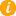Math K-Plus

# Number Two## What Makes Integer Number Two Unique?The integer number Two has many properties in mathematics. An integer is called even if it can be divided by 2. If a number ends in 0, 2, 4, 6, or 8 it means the whole number is even. The following are also properties of two.